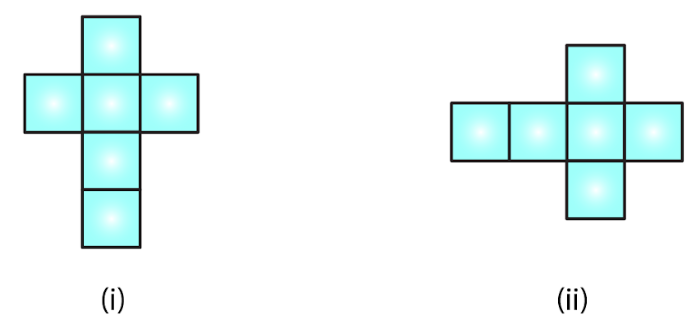# RD Sharma Solutions For Class 7 Maths Exercise 19.2 Chapter 19 Visualising Solid Shapes

Exercise 19.2 of Chapter 19 covers important topic nets for building three-dimensional figures along with illustrative examples to improve conceptual knowledge. Students who find it difficult in solving exercise wise problems of RD Sharma textbook can access PDF of solutions. For further reference, the students can download RD Sharma solutions for class 7 Maths. The problems are solved by our experts in the best possible way to make it easier for the students during exam preparation.

## Download the PDF of RD Sharma Solutions For Class 7 Maths Chapter 19 – Visualising Solid Shapes Exercise 19.2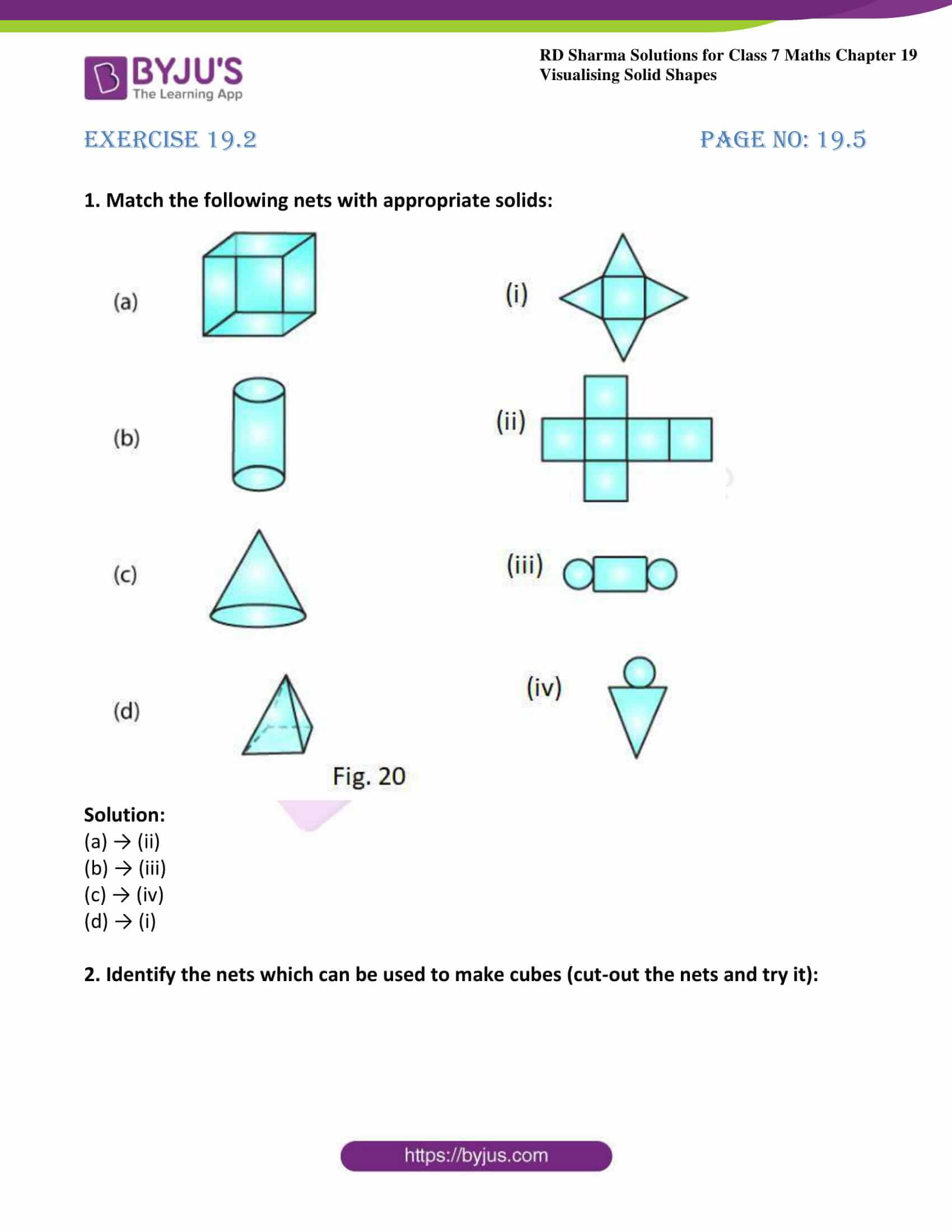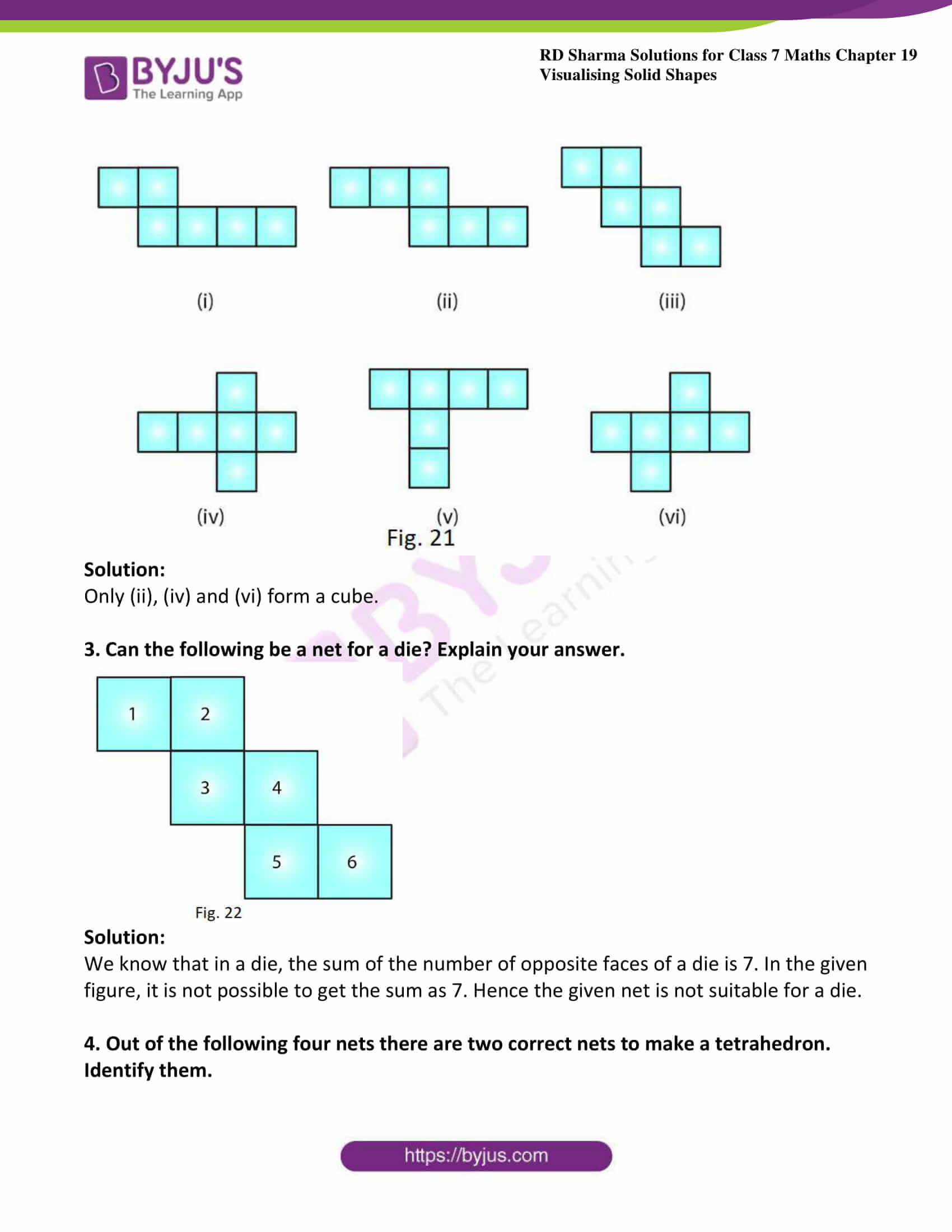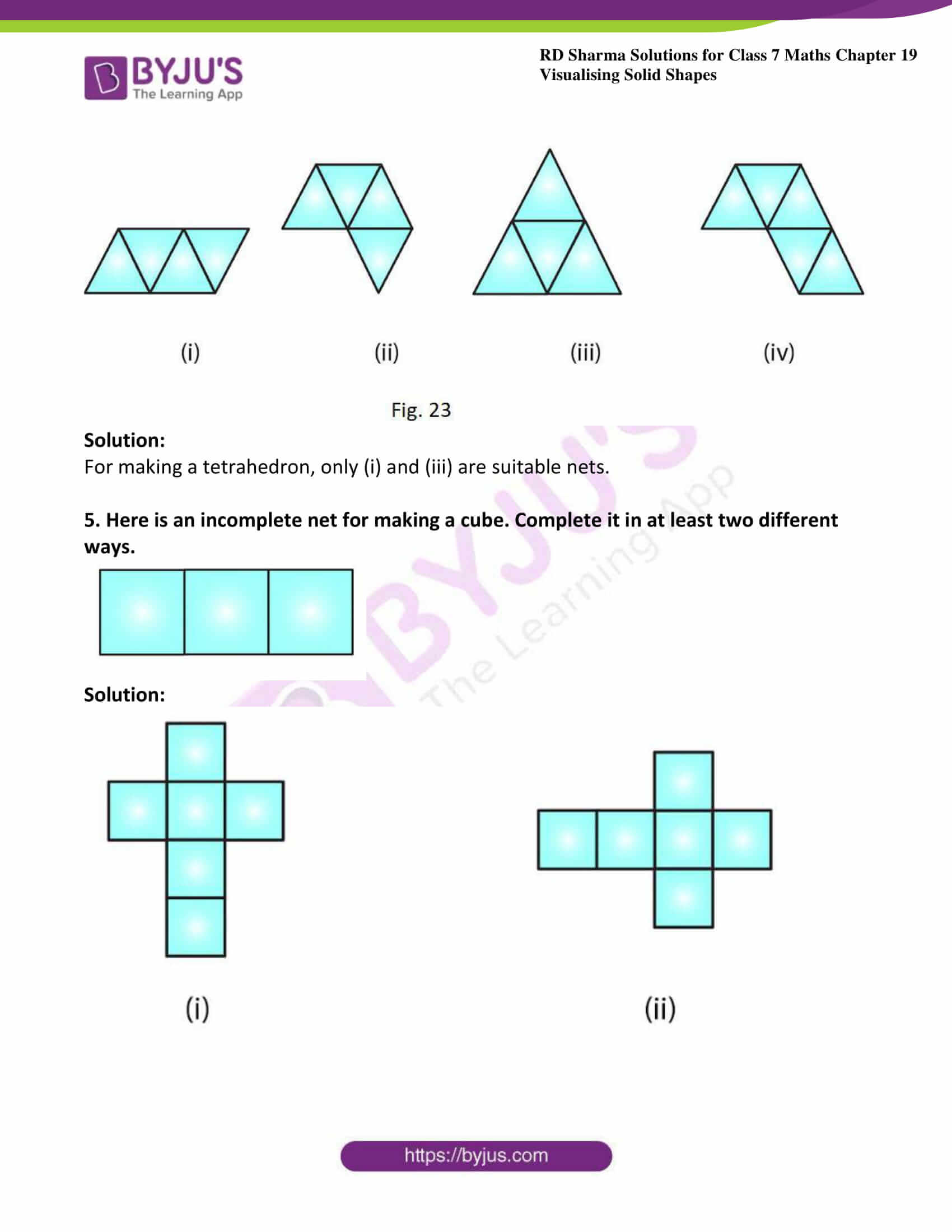### Access answers to Maths RD Sharma Solutions For Class 7 Chapter 19 – Visualising Solid Shapes Exercise 19.2

1. Match the following nets with appropriate solids: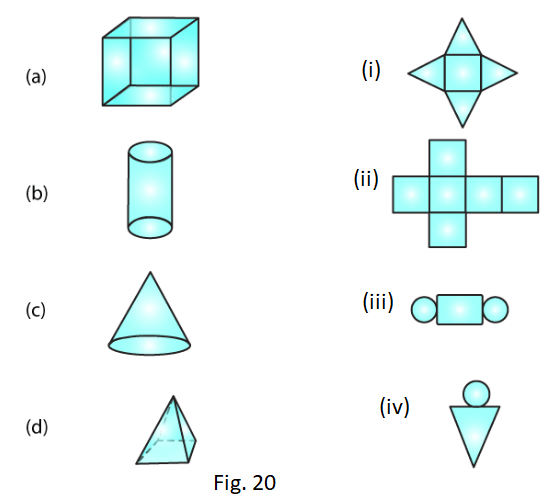Solution:

(a) → (ii)

(b) → (iii)

(c) → (iv)

(d) → (i)

2. Identify the nets which can be used to make cubes (cut-out the nets and try it):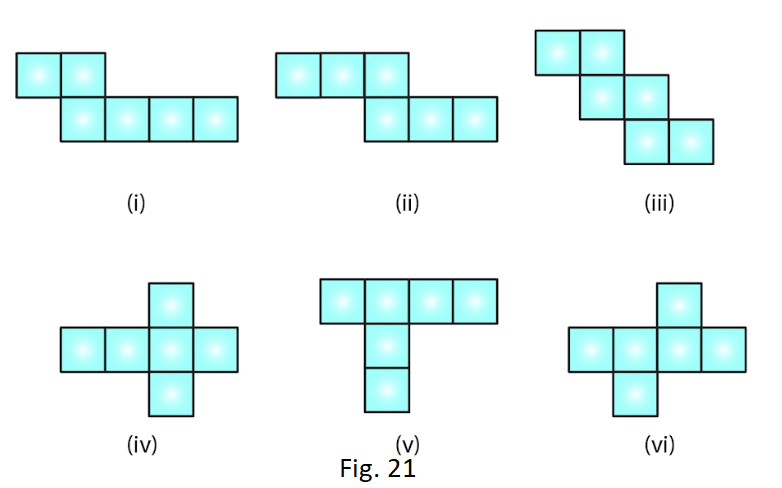Solution:

Only (ii), (iv) and (vi) form a cube.

3. Can the following be a net for a die? Explain your answer.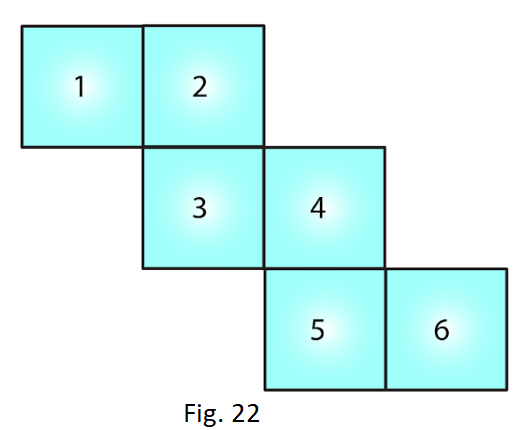Solution:

We know that in a die, the sum of the number of opposite faces of a die is 7. In the given figure, it is not possible to get the sum as 7. Hence the given net is not suitable for a die.

4. Out of the following four nets there are two correct nets to make a tetrahedron. Identify them.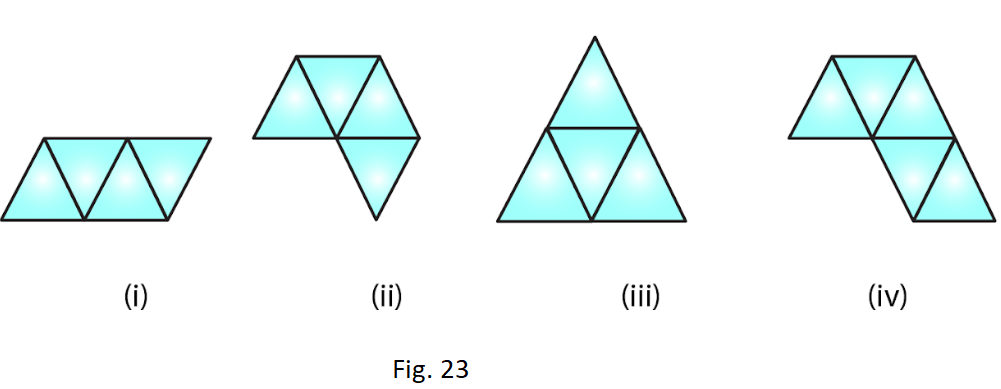Solution:

For making a tetrahedron, only (i) and (iii) are suitable nets.

5. Here is an incomplete net for making a cube. Complete it in at least two different ways.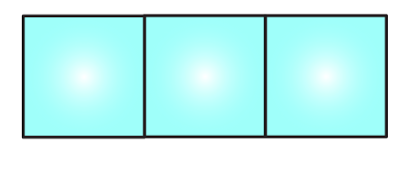Solution: